Hostname: page-component-cd4964975-xtmlv Total loading time: 0 Render date: 2023-03-31T16:29:22.525Z Has data issue: true Feature Flags: { "useRatesEcommerce": false } hasContentIssue true

# Integral Points on Elliptic Curves and Explicit Valuations of Division Polynomials

Published online by Cambridge University Press:  20 November 2018

## Abstract

HTML view is not available for this content. However, as you have access to this content, a full PDF is available via the ‘Save PDF’ action button.

Assuming Lang's conjectured lower bound on the heights of non-torsion points on an elliptic curve, we show that there exists an absolute constant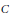$C$ such that for any elliptic curve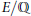$E/\mathbb{Q}$ and non-torsion point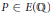$P\,\in \,E\left( \mathbb{Q} \right)$, there is at most one integral multiple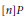$\left[ n \right]P$ such that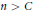$n\,>\,C$. The proof is a modification of a proof of Ingram giving an unconditional, but not uniform, bound. The new ingredient is a collection of explicit formulæ for the sequence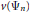$v\left( {{\Psi }_{n}} \right)$ of valuations of the division polynomials. For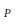$P$ of non-singular reduction, such sequences are already well described in most cases, but for$P$ of singular reduction, we are led to define a new class of sequences called elliptic troublemaker sequences, which measure the failure of the Néron local height to be quadratic. As a corollary in the spirit of a conjecture of Lang and Hall, we obtain a uniform upper bound on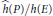$\widehat{h}\left( P \right)/h\left( E \right)$ for integer points having two large integral multiples.

## Keywords

Type
Research Article
Information
Canadian Journal of Mathematics , 01 October 2016 , pp. 1120 - 1158

## References

 Ayad, M., Points S-entiers des courbes elliptiques. Manuscripta Math. 76( 1992), no. 3-4, 305324.http://dx.doi.org/10.1007/BF02567763 CrossRefGoogle Scholar
 Baker, A., The Diophantine equation y2 = ax3 + bx2 + cx+ d. J. London Math. Soc. 43(1968), 19.http://dx.doi.Org/10.1112/jlms/s1-43.1.1 CrossRefGoogle Scholar
 Cheon, J. and Hahn, S., Explicit valuations of division polynomials of an elliptic curve. Manuscripta Math. 97(1998), no. 3, 319328.http://dx.doi.org/10.1007/s002290050104 CrossRefGoogle Scholar
 Cheon, J., The orders of the reductions of a point in the Mordell-Weil group of an elliptic curve. Acta Arith. 88(1999), no. 3, 219222.CrossRefGoogle Scholar
 Cornelissen, G. and Zahidi, K., Elliptic divisibility sequences and undecidableproblems about rational points. J. Reine Angew. Math. 613(2007), 133,http://dx.doi.Org/10.1515/CRELLE.2007.089 CrossRefGoogle Scholar
 David, S., Minorations déformes linéaires de logarithmes elliptiques. Mém. Soc. Math. France (N.S.) 62(1995).Google Scholar
 Einsiedler, M., Everest, G., Ward, T. , Primes in elliptic divisibility sequences. LMS J. Comput. Math. 4(2001), 113.http://dx.doi.Org/10.1112/S1461157000000772 CrossRefGoogle Scholar
 Ekedahl, T. , One semester of elliptic curves. EMS Series of Lectures in Mathematics, European Mathematical Society (EMS), Zürich, 2006.http://dx.doi.Org/10.4171/015 Google Scholar
 Everest, G. and Ward, T., The canonical height of an algebraic point on an elliptic curve. New York J. Math. 6(2000), 331342.Google Scholar
 Everest, G., Primes in divisibility sequences. Cubo Mat. Educ. 3(2001), no. 2, 245259.Google Scholar
 Everest, G. and King, H., Prime powers in elliptic divisibility sequences. Math. Comp. 74(2005), 20612071. http://dx.doi.org/10.1090/S0025-5718-05-01737-0 CrossRefGoogle Scholar
 Everest, G., Mclaren, G., and Ward, T., Primitive divisors of elliptic divisibility sequences. J. Number Theory 118(2006), no. 1,7189.http://dx.doi.Org/10.1016/j.jnt.2005.08.002 CrossRefGoogle Scholar
 Gezer, B. and Bizim, O., Elliptic divisibility sequences associated to elliptic curves with torsion points. 2011, arxiv:1101.3839 Google Scholar
 Everest, G., Squares in elliptic divisibility sequences. Acta Arith. 144(2010), no. 2, 125134. http://dx.doi.Org/10.4064/aa144-2-2 http://dx.doi.Org/10.4064/aa1 44-2-2 Google Scholar
 Hall, M. Jr., The Diophantine equation x3 — y2 = k. In: Computers in number theory (Proc. Sci. Res. Council Atlas Sympos. No. 2, Oxford, 1969), Academic Press, London, 1971, pp. 173198.Google Scholar
 Hindry, M. and Silverman, J. H., The canonical height and integral points on elliptic curves. Invent. Math. 93(1988), no. 2, 419450.http://dx.doi.org/10.1007/BF01394340 CrossRefGoogle Scholar
 Ingram, P., Multiples of integral points on elliptic curves. J. Number Theory 129(2009), no. 1, YEAR = 2009, 182208.http://dx.doi.Org/10.101 6/j.jnt.2008.08.001 http://dx.doi.Org/10.101 6/j.jnt.2008.08.001 CrossRefGoogle Scholar
 Ingram, P. and Silverman, J. H., Uniform estimates for primitive divisors in elliptic divisibility sequences. In: Number theory, Analysis and Geometry (In memory of Serge Lang), Springer-Verlag, Berlin, pp. 233263.Google Scholar
 Lang, S., Elliptic curves: Diophantine analysis. Grundlehren der Mathematischen Wissenschaften, 231, Springer-Verlag, Berlin, 1978.CrossRefGoogle Scholar
 Lang, S., Conjectured Diophantine estimates on elliptic curves. In: Arithmetic and geometry, I, Progr. Math., 35, Birkhâuser Boston, Boston, MA, 1983, pp. 155171.Google Scholar
 Mahé, V., Primepower terms in elliptic divisibility sequences. Math. Comp. 83(2014), no. 288, 19511991.http://dx.doi.org/10.1090/S0025-5718-2013-02790-1 http://dx.doi.org/10.1090/S0025-5718-2013-02790-1 CrossRefGoogle Scholar
 Pinter, A., On the magnitude of integer points on elliptic curves. Bull. Austral. Math. Soc. 52(1995), no. 2, pp. 195199. http://dx.doi.org/10.1017/S000497270001460X http://dx.doi.Org/10.1017/S0004972 70001460X CrossRefGoogle Scholar
 Schmidt, W. M., Integer points on curves of genus. Compositio Math. 81(1992), no. 1, 3359.Google Scholar
 Shipsey, R., Elliptic divisibility sequences.Ph.D. thesis, Goldsmith's College (University of London), 2000.Google Scholar
 Silverman, J. H., Lower bound for the canonical height on elliptic curves. Duke Math. J. 48(1981), no. 3, 633648. http://dx.doi.org/10.1215/S0012-7094-81-04834-1 CrossRefGoogle Scholar
 Silverman, J. H., A quantitative version ofSiegel's theorem: integral points on elliptic curves and Catalan curves. J. Reine Angew. Math. 378( 1987), 60100.Google Scholar
 Silverman, J. H., The difference between the Weil height and the canonical height on elliptic curves. Math. Comp. 55(1990), no. 192, 723743.http://dx.doi.org/10.1090/S0025-5718-1990-1035944-5 CrossRefGoogle Scholar
 Silverman, J. H., Advanced topics in the arithmetic of elliptic curves. Graduate Texts in Mathematics, 151, Springer-Verlag, New York, 1994.Google Scholar
 Silverman, J. H., The arithmetic of elliptic curves. Graduate Texts in Mathematics, 106, Springer, Dordrecht, 2009.Google Scholar
 Silverman, J. H. and Stange, K. E., Terms in elliptic divisibility sequences divisible by their indices. Acta Arith. 146(2011), no. 4, 355378.http://dx.doi.org/10.4064/aa146-4-4 http://dx.doi.Org/10.4064/aa146-4-4 CrossRefGoogle Scholar
 Silverman, J. H. and Stephens, N., The sign of an elliptic divisibility sequence. J. Ramanujan Math. Soc. 21(2006), no. 1, 117.Google Scholar
 Sprindzuk, V. G., Classical Diophantine equations. Lecture Notes in Mathematics, 1559, Springer-Verlag, Berlin, 1993.Google Scholar
 Stange, K., Elliptic nets and elliptic curves. Algebra Number Theory 5 (2011), no. 2, 197229. http://dx.doi.org/10.2140/ant.2011.5.197 http://dx.doi.org/10.2140/ant.2011.5.197 CrossRefGoogle Scholar
 Stark, H. M., Effective estimates of solutions of some Diophantine equations. Acta Arith. 24( 1973), 251259.CrossRefGoogle Scholar
 Stein, W. A., et. al., The Sage Development Team, Sage Mathematics Software (Version 4.6.2). 2011.http://www.sagemath.org Google Scholar
 Streng, M.,Divisibility sequences for elliptic curves with complex multiplication. Algebra Number Theory 2(2008), 183208. http://dx.doi.Org/10.2140/ant.2008.2.183. http://dx.doi.Org/10.2140/ant.2008.2.183 CrossRefGoogle Scholar
 Ward, M., Memoir on elliptic divisibility sequences. Amer. J. Math. 70(1948), 3174.http://dx.doi.Org/10.2307/2371930 CrossRefGoogle Scholar
 Yabuta, M., Primitive divisors of certain elliptic divisibility sequences. Experiment. Math. 18(2009), no. 3, 303310.http://dx.doi.ore/10.1080/10586458.2009.10129047 CrossRefGoogle Scholar